## Mathematics Paper 2 Questions and Answers - Butere Mock Exams 2021

INSTRUCTIONS TO CANDIDATES

1. Write your name, stream, admission number and index number in the spaces provided above.
2. The paper contains two sections, Section I and II
3. Answer all questions in section I and ONLY any FIVE questions from section II.
4. All answers and working must be shown on the question paper in the spaces below each question
5. Show all steps in your calculations, giving answers at each stage
6. Marks may be given for each correct working even if the answer is wrong
7. Non-programmable silent electronic calculators and KNEC mathematical tables may be used.

SECTION I
Answer all the questions in the spaces provided (50 marks)

1. The expression x2 + 10x + c + 2 = 0 is a perfect square. Find the value of c if it is a scalar. (2mks)
2. Muya was asked to truncate 7/9 to 3 significant figures. He rounded it off instead to 3 decimal places. Calculate the percentage error resulting from his rounding off. (3mks)
3. The co-ordinates of a point A is (2, 8, 3) and B is (-4, -8, -5). A point P divides AB externally in the ratio 7: -3.
Find the co-ordinates of P (3mks)
4. In a triangle XYZ, XY = 2cm, YZ (2√3-1) cm, and angle YXZ = 60º. Determine Sine XZY giving your answer in the form
m + √3, where M and N are integers (4mks)
n
5. Find the independent term of x in the expansion of (x32/X3)6 (3mks)
6. Solve for x: (log3x)2 – ½ log3 x= 3/2 (3mks)
7. The cash price of a T.V set is Ksh.13,800. Walter opts to buy the set on hire purchase terms by paying deposit of Ksh.2,280. If simple interest of 20% p.a is charged on the balance and the customer is required to pay by monthly installments for 2 years, calculate the amount of each installment. (3mks)
8. Make x the subject of the formula ax = 3r/2 - x2/2 (3mks)
9. Calculate the area under the curve y = 3x2 + 8 and bounded by lines; y = 0, x = 1 and x = 6, using the mid-ordinate rule with 5 strips. (3mks)
10. A circle is tangent to the y – axis and intersects the x- axis at (2,0) and (8,0). Obtain the equation of the circle in the form x2 + y2 +ax +by +c = 0, where a, b and c are integers (4mks)
11. A variable y varies as the square of x and inversely as the square root of z. Find the percentage change in y when x is changed in the ratio 5:4 and z reduced by 19% (3mks)
12. Solve for X in the equation:
2 Sin2x – 1 = Cos2x + Sin x, for 0º ≤ x ≤ 360º (3mks)
13. A die is biased so that when tossed, the probability of a narrator of a number n showing up, is given by p(n) = kn where k is a constant and n = 1, 2, 3, 4, 5, 6 (the numbers of the faces of the die)
1. Find the value of k (1mk)
2. If the die is tossed twice, calculate the probability that the total score is 11 (2mks)
14. In the figure below, the tangent ST meets chord VU produced at T. Chord SW passes through the Centre, O of the circle and intersects chord VU at X. Line ST = 12cm and UT = 8cm.1. Calculate the length of chord VU (1mk)
2. If VX : XU = 2 : 3, Find SX (2mks)
15. Dota measured the heights in centimeters of 104 trees seedlings are shown in the table below
 Height 0 - 19 20 - 29 30 - 39 40 - 49 50 - 59 60 - 69 70 - 79 No. of Seedlings 9 16 19 26 20 10 4
Calculate the quartile deviation (4mks)
16. Given that the ratio x:y = 2:3, find the ratio (5x – 2y) : (x +y) (2mks)

SECTION II
Answer ONLY five questions in this section (50 marks)

1. A curve is represented by the function, y = 2x3 + 3x2
1. Find:
1. the x-intercept of the curve (2mks)
2. the y-intercept of the curve (1mk)
2.
1. Determine the stationary points of the curve of the curve (3mks)
2. For each point in b(i) above, determine if it is maximum or minimum (2mks)
3. Sketch the curve in the space below (2mks)
2. Use ruler and a pair of compasses only in this question
1. Construct;
1. triangle ABC in which AB = 8.5cm, BC = 7.5cm and <BAC = 30º and <ABC = 105º (3mks)
2. a circle that passes through the vertices of triangle ABC. Measure the radius (3mks)
3. the height of triangle ABC with line AB as the base. Measure the height. (2mks)
2. Determine area of the circle that lies outside the triangle (2mks)
3.
1. Complete the table below, giving your values to 2 decimal places (2mks)
 x 0 30 60 90 120 150 180 210 240 270 300 330 360 (2cos x) -1 0 -2 -3 -2 -1 0 1 Sin x 0 1 0.50 0 -1 0
2. Draw the graph of y= (2 cos x) – 1 and y=sin x on the grid provided below. Use the scale 1cm represent 30º horizontal 2 cm represent 1 unit vertically and 2cm for 1 unit on the y-axis (4 mks)3. Use the graph to solve:
1. (2cos x) – 1 = -1.5 (1mk)
2. 2 cos x – sin x =1 (2mks)
4. State the amplitude of the wave y=2cos x – 1 (1mk)
4. A bag contains blue, green and red pens of the same type in the ratio 8:2:5 respectively. A pen is picked at random without replacement and its colour noted.
1. Determine the probability that the first pen picked is
1. Blue (1mk)
2. Either green or red. (2mks)
2. Using a tree diagram, determine the probability that
1. The first two pens picked are both green (4mks)
2. Only one of the first two pens picked is red. (3mks)
5. A and B are two points on the earth’s surface and on latitude 30ºN.The two points are on the longitude 40ºW and 104ºE respectively.
Calculate
1.
1. The distance from A to B along a parallel of latitude in kilometres. (3mks)
2. The shortest distance from A to B along a great circle in kilometre (4mks)
(Take π = 22/7 and radius of the earth =6370km)
2. If the local time at B is 8.00am, calculate the local time at A (3mks)
6. Lengths of 100 mango leaves from a certain mango tree were measured t the nearest centimeter and recorded as per the table below,
 Length in cm 9.5-12.5 12.5-15.5 15.5-18.5 18.5-21.5 21.5-24.5 No. of Leaves 3 16 36 31 14 Cumulative frequency
1. Fill in the table above. (2 mks)
2. Draw a cumulative frequency curve from the above data. (3 mks)
3. Use your graph to estimate
1. The quartile deviation of the leaves (3mks)
7. The number of leaves whose lengths lie between 13cm and 17cm. (2mks)
8. Use the trapezium rule with 7 ordinates to estimate the area enclosed by the curve y=½x2+3 and the lines x = 0, x = 6 and the x-axis. (4 mks)
9. Determine the exact area bounded the curve and the lines in section a) above (3 mks)
10. Calculate the percentage error from the trapezoidal rule (3 mks)
11. A manufacturer sells two types of books X and Y. Book X requires 3 rolls of paper while Book Y requires 2½ rolls of paper. The manufacturer uses not more than 600 rolls of paper daily in making both books. He must make not more than 100 books of type X and not less than 80 of type Y each day
12. Write down four inequalities from this information (4mks)
13. On the grid provided, draw a graph to show inequalities in (a) above (3mks)
14. If the manufacturer makes a profit of sh 80 on book X and a profit of sh 60 on book Y, how many books of each type must it make in order to maximize the profit. (3mks)## MARKING SCHEME

1. b2 = 4ac
52 = c + 2
25 = c + 2
c = 23
M1
A1
Correct expression in C
02
2. Truncated = 0.777
Rounded off = 0.778
A.E = 0.778 – 0.777 = 0.001
% E = x 100
= 0.12870012870012870012870012870013
B1
M1
A1
For both values correct
Expression for % Error
Allow 0.1287
03

3.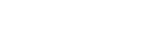P(-8.5, -20, -11)
M1
A1
B1
Expression
Correct matrix
Co-ordinate form
03

4.2
2√3 - 1
sinZ    sin60
2  2√3 - 1
sinZ    √3/2
sinZ =   √3    2√3 + 1
2√3 - 1    2√3 + 1
sinZ = 6 +√3
11
M1
M1
M1
A1
Correct substitution in sine rule
Surd form for sin 600
Correct attempt to rationalize
CAO
04
5. (x3)6 -6 (x3)5 + 15 (x3)(2/x3)2 - 20 (x3)3(2/x3)3
- 20 (x3)3(2/x3)3
- 20 x 8 = - 160
M1
M1
A1
Expansion up to the 4th term
Correct attempt to simplify
Constant term stated
03
6. Let log3x = y
2y2 – y – 3 = 0
(2y – 3)(y + 1) = 0
y = -1 or y = 1 ½
if log3x = -1, x = 3-1 = 1/3
if log3x = 1½ , x = 31.5 = 5.196
M1
A1
B1
For both correct
For both correct
03
7. P = cp – d = 13800 – 2280 = 11520
I = 11520 x 20 x 2/100= 4608
A = P + I = 11520 + 4608 = 16128
MI= 16128 ÷ 24
= 672
M1
M1 A1
Expression for simple interest
Expression for MI
8. 2ax + x2 = 3v
x2  + 2ax – 3v = 0
x2 + 2ax +a2 = 3v + a2
√(x + a)2 = √(3v +a2 )
x + a = ±√(3v +a2 )
x = -a ± √(3v +a2 )
M1
M1
A1
Completing the square
Correct attempt to solve
03
9.
 x 1.5 2.5 3.5 4.5 5.5 y 14.75 26.75 77.75 68.75 98.75
A= 1(14.75 +26.75+77.75+68.75+98.75)
= 253.75 square units
B1
M1
A1
Correct values of mid-ordinates
Expression for area
03

10.M(2 + 8 , 0 + 0) = M(5,0)
2         2
OA = OP = 5 units
AM = 5 – 2 = 3 units
OM = √(52 – 32 ) = 4 units
C(5,4) , r = 5
(x – 5)2 + (y – 4)2 = 52
x2 – 10x + 25 + y2 – 8y + 16 = 25
x2 + y2 -10x - 8y + 16 = 0
M1
M1
M1
A1
Expression for midpoint
Expression for OM
Correct substitution
Correct expanded form
04
11. y = kx2
√z
y1 =kx(5/4)2
√0.81
= 1.736k
% change =1.736 - 1 x 100
1
= 73.6%
M1
M1
A1
Correct substitution
Expression for percentage change
03
12. 3sin2x – sin x – 2 = 0
Let sin x = y
3y2- y -2 = 0
(3y + 2)(y – 1 ) = 0
y = 1 or y = -2/3
sin-1(1) = 90º
sin-1(-2/3) = 221.8º, 317.8º
x = 90º, 221.8º, 317.8º
M1
M1
A1
B1
Correct attempt to solve
For both
All values correct
04
13.
1. k + 2k + 3k + 4k + 5k + 6k = 1
21k = 1
k = 1/21
2. P(5&6) 0r P(6&5)
60
441
B1
M1
A1
Addition of probabilities (allow for any correct)
Allow 20/147
03
14.
1. Let VU = x
8(8 + x) = 122
8x = 144 – 64 =80
x = 10
2. VX = 2/5 x 10 = 4
XU = 3/5 x 10 = 6
XT = 6 + 8 = 14
SX = √(142 – 122) = 7.211
B1
M1
A1
x = 10
Expression for XT
03
15.
 h 10-19 20-29 30-39 40-49 50-59 60-69 70-79 f 9 16 19 26 20 10 4 cf 9 25 44 70 90 100 104
Q1 = 19.5 + 26 - 9 x 10 = 20.5625
26
Q3 = 49.5 + 78 - 70 x 10 = 53.5
20
Quartile deviation = 53.5 - 20.5625 = 16.46875
2
B1
M1
M1
A1
Cf
Q1 and Q3
Expression for quartile deviation
Allow 16.47
04
16. x/y2/3
x = 2/3y
5(2/3y) - 2y(2/3y) + y1
(4/3y):(5/3y) = 4:5
M1
A1
Correct substitution
02
17.
1.
1. x-intercept
x2(2x + 3) = 0
2. y-intercept
When x =0, y = 0
2.
1. Stationary points of curve = 0
dy/dx=6x2+ 6x = 0
6x (x + 1) = 0
x = 0 or x = -1
stationary points (0,0) and (-1,1)
2.
 x -2 -1 -0.5 0 1 dy/dx 12 0 -1.5 0 12 sketch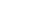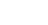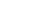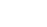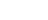maximum point (-1, 1), minimum point (0,0)
M1
A1
B1
M1
A1
B1
B1
B1
B1
Factorized form
Both correct
Both correct
Derivative equated to zero
Attempt to solve
For both
Checking points
For both
B1
B1

3.Points plotted (-1.5,0), (-1,1), (0,0)
Smooth curve
18.
1.
1.2. r = 5.2cm ± 0.1
3. h = 5cm ± 0.1
2. area of circle – area of triangle
= 84.98 – 21.25
= 63.73cm2
B1
B1
B1
B1
B1
B1
B1
B1
M1
A1
Construction of 300
Construction of 1050
Complete triangle, well labeled
Line bisectors
height dropped
follow through for r and h ± 0.1
10
19.
1.
 x 0 30 60 90 120 150 180 210 240 270 300 330 360 2cosx-1 1 1.73 -1 -2.73 -2.73 B1 0.73 1 sinx 0.5 0.87 0.87 -0.5 -0.87 -.087 0.5 0
2.3.
1. x = 102º or 253.5º ± 1.5
2. 2cos x -1 = sin x
x = 37.5º or 270º ± 1.5
3. Amplitude = 2
B1
S1
P1
C1
C1
B1
B1
B1
B1
Scale
Plotting for both
Smooth curve
20.
1.
1. 8/15
2. 2 + 5 =
15      152.
1. (8/155/15) + (8/155/15) + (2/15 x 8/15) + (2/15 x 5/15)
(5/15 x 8/15) + (5/15 x 2/15) = 132/225
2. P(BR) + P(GR) + P(RB) + P(RG)
(8/155/15) + (2/15 x 5/15) + (5/15 x 2/15) + (5/15 x 2/15) = 100/225
B1
M1
A1
B1
M1
M1
M1
A1
M1
M1
A1
Tree diagram draw with probabilities indicates
1 probability
probability
21.
1. Distance =  a  2πRcosθ
360
a=longitude difference
=40+140=180º
180 x 2 x 22 x 6370cos30
360          7
=17,337.8Km
2. θ=60 x 2
=1200
Distance =  θ   2πR
360
=120 x 2 x 22 x 6370
360          7
=13,346.7km
3. A(30ºN,40ºN)
B(30ºW,140ºE)
Difference in longitude=140+40
=180º
1º = 4min
180=?
180 x 4 = 720minutes
720 = 12hrs
60
8.00 + 12.00 = 20.00
=12.00hrs/8.00pm
10
B1
M1
A1
M1
A1
M1
A1
M1
M1
A1
For 180º
10
22.

1.  Length in cm 9.5-12.5 12.5-15.5 15.5-18.5 18.5-21.5 21.5-24.5 No. of leaves 3 16 36 31 14 cf 3 19 55 86 100
2.3.
1. Q3 = 19.25, Q1 = 17.15
½ (Q3 – Q1) = ½ (19.25 – 17.15)
= 1.05
2. 13cm - - 15.2, 17cm - - 15.8
15.8 – 15.2 = 0.3
B2
B1
S1
P1
C1
B1
M1
A1
B1
B1
All values correct
At least 4 values correct
Q3 & Q1 correct
Correct cf values
10
23.
 x 0 1 2 3 4 5 6 y 3 3.5 5 7.5 11 15.5 21
A = [(3 + 21) + 2(3.5 + 5 + 11 + 15.5)]
= 0.5[24 + 85]
= 54.5 sq.units

B2
M1
A1
M1
M1
A1
B1
M1
A1
10
24.
1. x ≤ 100
y ≥ 80
x ≥ 0
3x + 2½y ≥ 600
B1
B1
B1
B1

2.For each correct inequality
3. Objective function p = 80x + 60y
x = 200
y = 120
B1
B1
B1
B1
B1
B1
For each correct line drawn

• ✔ To read offline at any time.
• ✔ To Print at your convenience
• ✔ Share Easily with Friends / Students